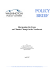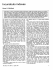# ACTA UNIVERSITATIS LODZIENSIS Piotr Wdowiński*, Aneta

## Transcription

ACTA UNIVERSITATIS LODZIENSIS Piotr Wdowiński*, Aneta
```ACTA
UNIVERSITATIS
LODZIENSIS
FOLIA OECONOMICA 192, 2005
P io tr W d o w iń ski* ,
A n e t a Z g l i ń s k a - P i e t r z a k **
TH E W ARSAW STOCK EXCHANGE INDEX WIG: M ODELING
AND FORECASTING***
Abstract. In this paper we have assessed an influence of the NYSE Stock Exchange
indexes (DJIA and NASDAQ) and European Stock indexes (D AX and FTSE) on the Warsaw
Stock Exchange index WIG within a framework of a GARCH model. By applying a procedure
of checking predictive quality of econometric models as proposed by Fair and Shiller (1990),
we have found that the NYSE market has relatively more power than European market in
explaining the WSE index WIG.
Keywords: Warsaw Stock Exchange, stock index, GARCH model, forecasting.
JEŁ Classification: C2, C5, C6, G l.
1. INTRODUCTION
T h e problem o f searching for the influence o f large m ark ets on others
has been well-know n from literature for years. H ow ever, this kind of
relationship has been widely studied through analyzing correlations between
individual indexes representing different m arkets (e.g. E rb et al. 1994; Bracker
and K o ch 1999).
In this paper we try to find such dependence between W IG index and
foreign stock m ark et indexes (D JIA , N A S D A Q and D A X , F TSE) by
estim ating param eters in regressions o f W IG .
T h e estim ates show which foreign stock m ark et affects Polish stock
exchange index m ore strongly. W e also test it by the forecasting approach
using forecast errors o f W IG index obtained in individual regressions. We
use also the idea o f com bined forecasts.
*
Dr (P h D ., Assistant Professor), Department of Econometrics, University of Łódź.
** Dr (Ph.D., Assistant Professor), Department of Econometrics, University of Łódź.
*** We are grateful to our colleagues from the Department o f Econometrics, University
of Łódź, and participants of FindEcon 2004 conference for helpful comments and suggestions
on earlier draft of the paper.
T here are m any m ethods used to com bine forecasts (e.g. C lem en 1989;
G ran g er 1989). As show n by Clemen and W inkler (1986), simple com ­
bination m ethods often work better than m ore com plex approach. The
aim o f com bining forecasts is to investigate w hether the forecast com ­
bin ation plays an im portant role in the im provem ent o f forecasting ac­
curacy. T he well-know n way o f com bining forecasts is to com pute linear
com bination o f forecasts generated by alternative m odels or obtained by
using different forecasting m ethods (e.g. Billio ct al. 2000, Claesscn and
M ittnik 2002).
D ependence between stock m arkets in different countries has been tested
for years. M any analyzes deal with m easuring correlation between returns
and diversified international portfolios (e.g. G rubel 1968, Levy and Sarnat
1970, A gm on 1972, Fiszeder 2003).
In the 1990s there appeared research o f how changes to stock prices
on one m ark et affect o th er m arkets (e.g. H am ao et al. 1990, K ing and
W adhw ani 1990, Engle and Susmel 1993, Fiszeder 2001).
T he focus o f this paper is to find the influence o f A m erican and
E u ropean indexes on W arsaw Stock Exchange index W IG . T here is a lot
o f research which prove th at this influence does exist. W e aim to examine
which m ark et - A m erican o r E uropean - has a stronger im pact on W IG
index.
T h e p aper is structured as follows. In Section 2 we give a brief overview
o f the G A R C H m ethodology. In Section 3 we test for influence o f foreign
stock indexes on W IG index. Com bined forecasting o f W IG index is applied
in Section 4, and finally we give concluding rem arks.
2. THE GARCH METHODOLOGY
M any m odels have been proposed to describe volatility o f returns. N ow
there is a com prehensive literature with several specifications o f autoregression
m odels. M any em pirical analyzes, however, have show n th a t G A R C H
ap p ro ach is the m ost appropriate. We also apply G A R C H m odeling in
this paper. T his is the m ost po p u lar class o f m odels used in m odeling the
financial tim e series o f high frequency (e.g. A kgiray 1989, Schwert and
Seguin 1990, N elson 1991, A ndersen et al. 1999, Osiewalski and Pipień
1999 and 2004, Bollerslev and W right 2000, Fiszeder 2001 and 2003,
Brzeszczyński and Kelm 2002, D om an M . and D om an R. 2003).
T he G A R C H m odel has been proposed independently by Bollerslev
(1986) and T ay lor (1986) as a generalization o f A R C H m odel introduced
by Engle (1982).
T h e m ain feature o f A R C H m odel is to describe the conditional variance
as an autoregression process. H owever, m ost em pirical tim e series require
using long-lag length A R C H m odels and a large num ber o f param eters
m ust be estim ated. T he solution o f the problem was G A R C H m odels which
gave better results (cf. Engle and Bollerslev 1986; N elson 1991).
T he basic linear generalized autoregressive conditional heteroscedastic
GARCHQ?, q) m odel is given as follows (e.g. Bollerslev 1986):
(1)
Уг = x (k)l®k + ev
where:
(2)
e, = 9, %/h t,
and h, is a function o f conditional variance represented as:
(3)
h,
= y0 + X
i=i
YiŹ-i +
Z
Vj K - j,
i
where: 3, is i.i.d. with £ (9 ,) = 0 and v a r ( 9 , ) = l , yo >0*
p
( p ^ 0. If
я
£ y i + Y j (Pj< 1* ^ e process h, is covariance stationary.
i=i
j= i
Engle and Bollerslev (1986) considered also G A R C H
processes with
X ľ i + Y j V j — Ь which they denoted integrated G A R C H (IG A R C H ).
In em pirical research the m ost frequently used is G A R C H (1, 1) m odel
in w hich h, = y 0 + y iE ? - i + <Piht ~ i a n ^ Уо >
Уi ^
9 > i^ 0 . W hen
У\ + (P\ < 1, th an unconditional variance o f et is given by var (e,) = .
1
1
i-Y i-V i
T he coefficients o f the m odel are then easily interpreted, w ith the estim ate
o f yt show ing the im pact o f current news on the conditional variance
process and the estim ate o f <pt as the persistence o f volatility to a shock
or, alternatively, the im pact o f “ old ” news on volatility.
Recently a num ber o f new form ulations have been proposed, for example
exponential G A R C H (p, q) m odel (E G A R C H , e.g. N elson 1991), G JR G A R C H m odel (e.g. G losten et al. 1993), G A R C H in the m ean (G A R C H M(p, q), e.g. Engle et al. 1987), power G A R C H (P G A R C H , e.g. D ing et
al. 1993), and the fractionally integrated G A R C H (F IG A R C H , e.g. Baillie
et al. 1996).
Such m odels are com m only applied in financial tim e series research. The
estim ation o f G A R C H m odels is bo th classic and non-classic, e.g. Bayesian
ap p ro ach (e.g. Osiewalski and Pipień 1999, 2004).
In Section 3 we test m odels for influence o f foreign stock m arkets on
the Polish m arket.
3. TESTING FOR INFLUENCE OF FOREIGN STOCK INDEXES ON WIG INDEX
In the p ap er we use a G A R C H m ethodology with G A R C H (1,1) models.
We have found th at both A R C H and G A R C H effects appeared to be
statistically significant in the dependence tested.
T he focus o f the paper is to find the influence o f A m erican and European
stock m ark et indexes on W IG index over the period 1.01.1995 to 29.12.2003
(2346 observations).
A fter introducing suggested foreign indexes to the G A R C H (1, 1) equation
describing W IG index, it was im possible to separate m utual relationships
between index W IG and foreign indexes, m ainly D A X and F T SE , because
the estim ates used to be statistically insignificant and had opposite signs
which negated the assum ptions o f positive influence o f the biggest stock
m ark ets on index W IG . Hence, we were unable to include both European
indexes D A X and FT SE in one equation, and we decided to test for the
influence o f E u ro p ean m arkets by two equations accordingly.
In tu rn , we analyze the following models:
• for A m erican m arket:
•
(4)
•
(5)
•
•
wigt = a 0 -f a ^ j i a , - t + tx2nasdaq,^l + e(,
for E u ro pean m arket:
•
•
wig, = ot0 + tx ^a x , _ x + e(,
or
(6)
•
•
wig, = a 0 + c tjts e t- i + e„
where the variables are first differences o f natural logarithm s, so they are
the close-to-close returns on corresponding indexes.
T h e estim ation was m ade by m axim um likelihood m e th o d 1 for daily
d ata from 1.01.1995 to 29.12.2003.
T he equations (4), (5) and (6) were recursively estim ated and helped to
o b tain a series o f 250 one-period-ahead quasi ex-ante forecasts. T he estimates
1 We used Econometric Views package.
o f individual equations were generally statistically significant. T he sum у1 + ф1
in the eq u atio n o f conditional variance was around 0.92, while the estim ates
were ab o u t 0.12 and ф1 ab o u t 0.80. Hence, we can conclude th at the
im pact o f cu rren t news on volatility in the conditional variance process is
sm aller th a n the im pact o f “ old ” news.
T he coefficient o f determ ination R -squared for corresponding equations
(4), (5) and (6) was ab o u t 12%, 3% and 3% respectively.
In T able 1 we give m easurem ent o f ex-post errors2 for forecasts obtained
from respective equations o f index W IG .
Table 1. Ex-post errors o f index WIG forecasts
Specification
DJIA-NASDAQ
DAX
FTSE
MAE
0.00922
0.00926
0.00929
RMSE
0.01193
0.01197
0.01213
0.75195
0.82760
0.84897
n
0.005
0.004
0.005
n
0.411
0.575
0.582
n
0.588
0.425
0.417
THEIL
T P\ (%)
19.69
13.39
15.75
TP2 (%)
50.21
43.57
47.72
In the analysis o f ex-post errors we used turn in g points test statistic
- T P 1 - represented as:
n\
T P I = N ( y * > 0 л r t-ir ,* -! > 0 |у , - 1 < 0)
N (rtr , - i < 0 )
where:
N (rtrt_ 1 < 0) - a num ber o f turning points in em pirical series;
N (r tr* > 0 л r,_ t r*_! > 0 |r rr,_ i < 0) - a num ber o f po in ts, in which
changes to direction in em pirical and forecasting series are the sam e in the
period t and t — 1 under the condition th at the points are turning points
in em pirical series.
W e also used the following direction quality m easure (e.g. Welfe and
Brzeszczyński 2000):
2
We used the following ex-post errors: MAE - mean absolute error, RMSE - root mean
square error, THEIL - Theiľs inequality coefficient, /1, 12, 13 - decomposition of Theil’s
inequality coefficient, TP 1 and TP2 - turning points test statistics.
N (r ,r * > 0 )
(8)
TP1 = N
ш(r,r, * 0 )
where:
N (rtr* > 0) - a num ber o f points in which direction changes in em pirical
and forecasting series are the same.
T he m ost accurate forecasts we obtained from the equation with American
indexes. F o recasts basing on E uropean indexes, however, were close to each
o th er as far as errors are concerned.
In Section 4 we apply a m ethodology o f com bined forecasting. We test
for relative im portance o f foreign stock m arkets in explaining W IG index.
4. COMBINED FORECASTS OF WIG INDEX
In o rd er to assess the pow er o f influence o f foreign m arkets on the
Polish m ark et we estim ated the following F air and Shiller (1990) equation
(e.g. W dow iński 2004):
(9)
y , ~ y t-1 =
+
yi - i) + <*2(t-iý2t - yt- i ) + et
where
- denotes one-period-ahead forecasts o f y, generated by the
m odel 1, i.e. the m odel w ith A m erican indexes based on inform ation
available up to the m om ent t — 1 with the use o f recursive estim ation for
each period t. T he predictor , - i ý 2t - denotes one-period-ahead forecasts
g enerated accordingly by the m odel 2, i.e. th e m odel w ith E uropean
indexes, while e is an erro r term , e ~ /N (0 , o f). If neither m odel 1, nor
m odel 2 co ntain any relevant inform ation in term s o f forecasts quality for
variable у in period t, the estim ates o f
and a 2 will be statistically
insignificant. If both m odels generate forecasts th at contain independent
in form ation, the estim ates o f a.l and a 2 should both be statistically sig­
nificant. If b oth m odels contain inform ation but inform ation contained in
forecasts generated by m odel 2 is completely contained in forecasts gene­
rated by m odel 1 and furtherm ore m odel 1 contains additional relevant
in form ation, the estim ate o f ol1 will be statistically significant while the
estim ate o f a 2 statistically insignificant. If both forecasts contain the same
inform ation, they are perfectly correlated and the estim ation o f param eters
o f (9) is n o t possible.
Because th e influence o f E u ro p e an m ark e ts was described by two
equations, we estim ated m odel (9) for tw o cases:
• for em pirical W IG index and forecasts generated by (4) and (5).
• for em pirical W IG index and forecasts generated by (4) and (6).
Below in T ab le 2 we present estim ation results o f equation (9).
Table 2. Estimation results of Fair and Shiller equation
In te r­
cept
D JIA -N A S D A Q
DAX
FT S E
0.0007
1.0018
0.5495
2.105
0.3989
1.4923
X
J
0.0007
1.0226
0.6523
3.1112
J
i
0.2915
1.3777
W ald
(U SA )
W ald
(E U R )
0.0119
0.2211 0.3888
4.4313
0.6407
2.0719
(0.6382) (0.4214) (0.0353)
(0.7258)
(0.1356)
0.0119
0.3223 4.073
0.8254
9.6799
2.0818
(0.5702) (0.3961) (0.0019)
(0.6618)
1.8982
(0.1683)
s.
J-B
D-W
ARCH WHITE
2.227
R2
Obs.
0.4429
250
0.4422
250
W ith italics we have denoted i-statistics with regard to estimates. Respective
test probabilities with regard to test statistics are given in brackets. We
applied the following tests: Jarque-B era norm ality o f residuals test (J-B),
conditional heteroscedasticity test (A R C H ), W hite’s test for heteroscedasticity
(W hite), W ald coefficient restrictions test (W ald). T he D -W stands for
D u rb in -W atso n test statistic.
T he test statistics and their probabilities in case o f J-B, D -W , A R C H
and W hite’s tests denote th at errors in both m odels are n orm al, with no
au to co rrelatio n , no A R C H effects and no heteroskedasticity. T hus we can
test the pow er o f influence o f foreign indexes on W IG index using tstatistic.
It can be easily seen th at the influence o f A m erican m ark e t indexes
D JIA and N A S D A Q turned ou t to be m ore relevant for W IG index than
E u ro p ean indexes D A X and F T SE . It is supported by the significance of
estim ates and their size. W e can conclude, th at inform ation contained in
forecasts generated by m odels (5) o r (6) is fully contained in forecasts by
m odel (4). Therefore, A m erican indexes D JIA and N A S D A Q were m ore
influential th an E uropean indexes (D A X or F T S E ) as regards W IG index.
T his conclusion is also confirm ed by W ald coefficient restrictions test, i.e.
we should reject the null th at the respective coefficient equals zero in case
o f m odel (4) and should not reject the null in case o f m odels (5) or (6).
Sim ilar conclusions about the co-dependence o f m arkets in case o f Poland
were d raw n by e.g. Fiszeder (2003).
Since the p aram eters in equation (9) do n o t sum up to one, we should
n o t tre a t them as weights in calculating com bined forecasts.
T herefore, to calculate the weights, we used the nonlinear program m ing
problem (NLP):
m in /(co) = <o7 Vm,
( 10)
ю г1 = 1,
to > 0,
where:
ю =
Г( o . l
- is the vector o f weights;
La>2\
V - the variance-covariance m atrix o f forecast errors.
In T able 3 we present covarianccs and correlations o f forecasts errors.
Table 3. Covariance and correlation coefficients matrix of forecast errors
Covariance
Specification
DJIA-NASDAQ
DAX
FTSE
DJIA-NASDAQ
DAX
FTSE
0.0001417
0.0001380
0.0001375
0.0001427
0.0001427
0.0001427
Correlation
DJIA-NASDAQ
DAX
FTSE
1
0.9708
0.9546
1
0.9875
1
A fter solving the problem o f nonlinear program m ing (10) we obtained
the follow ing weights which are given in T able 4.
Table 4. The weights in a NLP problem
DJIA-NASDAQ
DAX
FTSE
0.5664
0.4336
X
0.6734
X
0.3266
As we can notice the weights are close to estim ated param eters in
equation (9). Thus we confirmed earlier conclusions about the strong influence
o f A m erican m arket on the Polish stock m arket. As show n in T able 5, we
could n o t substantially reduce the variance o f com bined forecast errors,
because o f the high and positive correlation between forecast errors from
individual m odels (4), (5) and (6).
Table 5. Variance of forecast errors
Individual forecast
0.0001417
0.0001427
0.0001427
DJIA-NASDAQ
DAX
FTSE
Combined forecast
0.0001406
0.0001409
DJIA-NASDAQ-DAX
DJ1A-NASDAQ-FTSE
A fter calculating optim al weights, we calculated com bined forecasts and
assessed th eir accuracy. T he results are given in T ab le 6.
Table 6. Ex-post errors of combined forecasts of WIG index
Specification
DJIA-NASDAQ
MAE
0.00918
0.00916
RMSE
0.01186
0.01188
THEIL
0.78937
0.79123
n
0.005
0.005
4
0.514
0.515
4
0.537
0.534
DJIA-NASDAQ-FTSE
TP\ (%)
15
17
TP2 (%)
48.55
48.96
It is clearly
obtained from
Finally, we
indexes o f both
equations:
show n th a t com bined forecasts are n o t superior to forecasts
each m odel separately.
tested the forecasting quality o f the equations including
foreign m arkets at the same time. We estim ated the following
(11)
•
•
•
•
wig, = a 0 + ctydjia,-1 + &2nasdaq,-! + a 3dax,_ i + e,
(12)
wig, = ß 0 + ß l d jia ,-l + ß 2nasdaqt- i + ß 1f t s e t- 1 + Zt.
•
•
•
•
T h e estim ates o f a 3 and ß 3 describing the influence o f E u ro p ean m arket
were usually insignificant. Also, as before, we obtained opposite signs o f
estim ates which does not com ply with the postulate o f positive influence
o f the biggest stock m arkets on W IG index.
By applying the all-index approach itself we were no t able to determ ine
the pow er o f influence o f individual m arkets on the Polish m arket due to
high correlatio n coefficients between indexes and difficulties in estim ating
the param eters. Therefore, the com bined forecasts approach suggested in
the paper can be considered correct.
D espite questionable statistics in results o f regressions (11) and (12) and
their weak econom ic properties we used them to forecast W IG index. The
errors o f these forecasts are shown in T able 7.
Table 7. Ex-post errors of forecasts obtained in all-index equations
Specification
DJIA-NASDAQ-DAX
DJIA-NASDAQ-FTSE
MAE
0.00918
0.00932
RMSE
0.01191
0.0123
THEIL
0.75361
0.7531
n
0.005
0.005
n
0.42
0.394
4
0.579
0.606
TP 1 (%)
21.26
19.69
TP2 (%)
51.04
50.21
A s in the case o f com bined forecasts they are no t o f better quality than
forecasts obtained from individual m odels separately.
5. CONCLUSIONS
In this p ap er we attem pted to assess the influence o f A m erican and
E u ro p ean stock m arkets on the Polish stock m arket. In o u r analysis we
used the G A R C H m ethodology which is popular to describe the financial
phenom ena o f high frequency. We have found th at the situation on Am erican
m ark et had m ore influence on the Polish m arket than the situation on
E u ro p ean m ark ets in the analysed period during 1.01.1995 - 29.12.2003.
We based these conclusions on the analyzis o f forecasting equations for
W IG index and on the analysis o f com bined forecasting. O u r results are
consistent w ith those obtained by other authors for the Polish stock m arket
(e.g. Fiszeder 2001 and 2003; Brzeszczyński and K elm 2002).
W e should notice, however, a few aspects which can affect conclusions
d raw n from this kind o f research.
F irstly, it m ust be rem em bered th at there is a strong co-dependence
am ong the biggest stock m arkets so it is difficult to show the im pact of
one individual m ark et on another one in a sim ple approach.
Secondly, it is very im portant to choose the proper indexes as determ inants
o f m ark et developm ent. It is necessary, then, to test also the influence of
o th er indexes and stock m arkets - including em erging m arkets - on the
Polish m arket.
T hirdly, the Polish stock m arket m ay be treated by foreign investors as
one o f secondary im portance, and consequently dom estic investm ent funds
m ay influence the variability o f returns m ore than situation on foreign
m arkets.
In th e p ap er we have used only series o f 250 one-period-ahead forecasts.
In fu tu re research we will attem pt to show how the param eters reflecting
the influence o f A m erican stock m ark et and o ther m arkets on the Polish
m ark et change over time.
REFERENCES
Agmon, T. (1972), “The Relations Among Equity Markets in the United States, United
Kingdom and Japan”, Journal o f Finance, 28, 839-855.
Akgiray, V. (1989), “Conditional Heteroskedasticity in Time Series of Stock Returns: Evidence
and Forecasts”, Journal o f Business, 62, 55-80.
Andersen, T. G., Bollerslev, T. and Lange, S. (1999), “Forecasting Financial Market Volatility:
Sample Frequency vis-a-vis Forecast Horizon”, Journal o f Empirical Finance, 6, 457-477.
Baillie, R. T., Bollerslev, T. and Mikkelsen, H. O. (1996), “Fractionally Integrated Generalized
Autoregressive Conditional Heteroskedasticity”, Journal o f Econometrics, 74, 3-30.
Billio, M., Sartore, D. and Toffano, C. (2000), “Combining Forecasts: Some Results on
Exchange and Interest Rates”, The European Journal o f Finance, 6, 126-145.
Bollerslev, T. (1986), “Generalized Autoregressive Conditional Heteroskedasticity”, Journal o f
Econometrics, 31, 307-327.
Bollerslev, T. and Wright, J. H. (2000), “Semiparametric Estimation of Long-memory Volatility
Dependencies: The Role o f High-frequency Data”, Journal o f Econometrics, 98, 81-106.
Bracker, К. and Koch, P. D . (1999), “Economic Determinants o f the Correlation Structure
Across International Equity Markets”, Journal o f Economics and Business, 51, 443-471.
Brzeszczyński, J. and Keim, R. (2002), Ekonometryczne modele rynków finansowych. Modele
kursów giełdowych i kursów walutowych (Econometric Models o f Financial Markets. Models
of Stock Prices and Exchange Rates), Warszawa: WIG-Press.
Claessen, H. and Mittnik, S. (2002), “Forecasting Stock Market Volatility and the Informational
Efficiency of the DAX-Index Options Market”, The European Journal o f Finance, 8, 302-321.
Clemen, R. T. (1989), “Combining Forecasts: A Review and Annotated Bibliography”,
International Journal o f Forecasting, 5, 559-583.
Clemen, R. T. and Winkler, R. L. (1986), “Combining Economic Forecasts” , Journal o f
Business and Economic Statistics, 4, 39-46.
Ding, Z., Granger, C. W. J. and Engle, R. F. (1993), “A Long Memory Property of Stock
Market Returns and a New M odel”, Journal o f Econometrics, 73, 185-215.
Doman, M. and Doman, R. (2003), “Prognozowanie dziennej zmienności indeksu WIO
określonej za pomocą danych o wyższej częstotliwości” (Forecasting Daily Volatility of
WIG Index with Higher Frequency Data). In: Milo, W. and Wdowiński, P. (eds.)
Współczesne metody analizy i prognozowania finansowych rynków kapitałowych (New
Methods of Analysis and Forecasting Financial Markets), Acta Universität ú Lodziensis,
Folia Oeconomica, 166.
Engle, R. F. (1982), “Autoregressive Conditional Heteroscedasticity with Estimates o f the
Variance of the United Kingdom Inflation”, Econometrica, SO, 987-1008.
Engle, R. F. and Bollerslev, T. (1986), “Modeling the Persistence o f Conditional Variance”,
Econometric Reviews, 5, 1-50.
Engle, R. F., Lilien, D. M. and Robins, R. P. (1987), “Estimating Time Varying Risk Premia
in the Term Structure: The ARCH-M Model”, Econometrica, 55, 391-407.
Engle, R. F. and Susmel, R. (1993), “Common Volatility in International Equity Markets”,
Journal o f Business and Economic Statistics, 11, 167-176.
Engle, R. and Kroner, K. (1995), “Multivariate simultaneous GARCH”, Econometric Theory,
11, 122-150.
Erb, С. В., Harvey, C. R. and Viskanta, T. E. (1994), “F'orecasting International Equity
Correlations”, Financial Analyst Journal, November-December, 32-45.
Fair, R. C. and Shiller, R. J. (1990), “Comparing Information in Forecasts from Econometric
Models”, The American Economic Review, 80, 375-389.
Fiszeder, P. (2001), “Zastosowanie modeli GARCH w analizie krótkookresowych zależności
pomiędzy warszawską giełdą papierów wartościowych a międzynarodowymi rynkami akcji”
(GARCH Modelling o f Short-term Co-dependence Between the Warsaw Stock Exchange
and International Stock Markets), Przegląd Statystyczny, 48, 345-364.
Fiszeder, P. (2003), “Testy stałości współczynników korelacji w wielorównaniowym modelu
GARCH - analiza korelacji między indeksami giełdowymi: WIG, DJIA i NASDAQ
COMPOSITE” (Stability Tests of Correlation Coefficients in Multivariate GARCH Model
- An Analysis o f Correlation Between Stock Indexes: WIG, DJIA and NASDAQ
COMPOSITE), Przegląd Statystyczny, 50, 53-71.
Glosten, L. R., Jagannathan R. and Runkle, D . E. (1993), “On the Relation Beet wen the
Expected Value and Volatility of the Nominal Excess Return on Stocks”, Journal o f
Finance, 48, 1779-1801.
Granger, C. W. J. (1989), “Invited Review: Combining Forecasts - 20 Years Later”, Journal
o f Forecasting, 8, 167-173.
Grubel, H. (1968), “Internationally Diversified Portfolios: Welfare Gains and Capital Flows”,
American Economic Review, 58, 1299-1314.
Hamao, Y., Masulis, R. W. and Ng, V. (1990), “Correlations in Price Changes and Volatility
Across International Stock Markets”, The Review o f Financial Studies, 3, 281-307.
King, M. A. and Wadhwani, S. (1990), “Transmission of Volatility Between Stock Markets”,
The Review o f Financial Studies, 3, 5-33.
Levy, J. and Samat, M. (1970), “International Diversification of Investment Portfolios”, American
Economic Review, 60, 668-675.
Nelson, D. (1991), Conditional Heteroscedasticity in Asset Returns: A New Approach”,
Econometrica, 59, 347-370.
Osiewalski, J. and Pipień, M. (1999), “ Estymacja modeli GARCH: MNW i podejście
bayesowskie” (Estimation of GARCH Models: ML and Bayesian Methods), Przegląd
Statystyczny, 46, 2.
Osiewalski, J. and Pipień, M. (2004), “ Bayesian Comparison of Bivariate ARCH-Type
Models for the Main Exchange Rates in Poland”, Journal o f Econometrics, 123 (2),
371-391.
Schwert, G. W. and Seguin, P. J. (1990), “ Heteroskedasticity in Stock Returns”, Journal o f
Finance, 13, 838-851.
Taylor, S. J. (1986), Modeling Financial Time Series, Chichester, Wiley.
Wdowiński, P. (2004), Determinants o f Country Beta Risk in Poland, CESifo Working Paper, 1120.
Welfe, A. and Brzeszczyński, J. (2000), “Direction Quality Measures for ARCH Models: The
Case of Warsaw Stock Exchange Stock Prices”, ln: Welfe, W. and Wdowiński, P. (eds.),
Macromodels ’99, Conference Proceedings, Łódź: Absolwent.
P io tr W dow iński, A n eta Z g liń sk a -P ietrza k
MODELOWANIE I PROGNOZWANIE INDEKSU WIG
(Streszczenie)
W artykule podjęliśmy próbę oceny wpływu indeksów rynku amerykańskiego DJIA
i NASDAQ oraz indeksów rynku europejskiego DAX i FTSE na indeks WIG z giełdy
w Warszawie. D o modelowania tego wpływu wykorzystaliśmy metodologię GARCH. Stosując
metodologię łączenia prognoz oraz metodologię oceny jakości prognostycznej modeli ekono­
metrycznych, zaproponowane w pracy Fair i Shiller (1990), pokazaliśmy, że rynek NYSE ma
względną przewagę nad rynkiem europejskim w wyjaśnieniu zmian indeksu WIG.
```

### agricultural services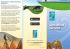### 3bMeteo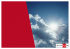### Vitalii Shpyg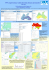### pdf page 2### Heart and sole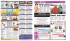### Extra support for crazy ponytails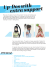### Regional Growth Forecasts 2014-2040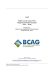### Jan 07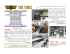### Financial Results Briefing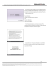### Where Will US Population Growth Occur? A Glimpse at### AccuWeather Enterprise Solutions Overview